# Zener Max Current Iz

#### SamR

Joined Mar 19, 2019
4,911
I have a 1W 1N4736A 6.8Vz diode powered by a 12VDC supply.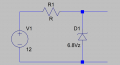From the PDF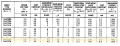Which tells me Max Iz is 133mA. So why wouldn't it be A=W/Vs-Vz = 1W/12Vs-6.8Vz = 1W/5.2Vo = 192mA? Is it because they are using T=50°C?

EDIT: Correct zener orientation.

Last edited:

#### Audioguru again

Joined Oct 21, 2019
6,428
1) A zener diode should be reverse biased. Yours is forward biased.
2) 5.2V is across the resistor that is powering your zener diode. The zener diode is 6.8V and with its max 133mA of current it heats with 0.9W. Don't overload it so it fries. Have you even been in an ambient temperature of 50 degrees C (122 degrees F)?

#### DickCappels

Joined Aug 21, 2008
10,102
In other words, the maximum current may be determined by dividing the Zener's power rating by the Zener voltage and allowing a substantial safety margin.

@Audioguru again When I was young and rode a motorcycle to work I would arrive on winter mornings, take of my jacket and throw open the door to the 55° heat tank use to improve field reliability in out product. It was heaven.

#### SamR

Joined Mar 19, 2019
4,911
1) A zener diode should be reverse biased. Yours is forward biased.
Ooops, that was my bad sketching the circuit quickly to put here.

The Zener is not dropping 6.8V, so it's 5.2V x 133mA Iz Max = ~0.7W. That leaves a lot of overhead or derating for temp.

Where I am confused is in determining the value of R with a load attached. Without the load, R should limit the Zener to less than Iz Max. One book gives a figure of using a value of 5mA for Iz and another 100mA Iz for the circuit under load. So how much current is left to use for load resistance and for the Resistor? Apparently calculating Iz Max from the 1W rating isn't the answer since the PDF gives a different value.

So if I use 133mA Max for R without a load, that gives me V/I = R = 12V/0.133A = ~90Ω. So, depending on what current I allow for the Zener that leaves me ~33-128mA for the load? Am I going on the right path here?

#### MrChips

Joined Oct 2, 2009
29,791
Let's see if I can paint a simpler picture.

R is a series resistor that supplies current to a load.
Think of the load as being the zener diode in parallel with a true load resistor.
You select the max load current Imax. The job of the zener is to clamp the voltage at the zener voltage Vz.

Now you can calculate the series resistance:
R = (Vs - Vz) / Imax
where Vs is the supply voltage and Vz is the zener voltage.
Calculate the power dissipation of R:
P = Imax x Imax x R
Choose a resistor with a power rating 1.5 - 2 times P.

Here is the scoop. R has to supply Imax all the time and dissipate P watts all the time.
If the true load is drawing Imax, then the zener diode gets 0A and is just there for the ride.
If the load takes anything less than Imax the zener diode has to shunt the rest to ground.
If the load takes 0A then the zener diode has to shunt Imax. It is always a total of Imax current.

Now you can calculate the worse case (max) power dissipation of the zener diode:
Pz = Imax x Vz
Choose a zener diode with a power rating at 1.5 - 2 times Pz.

Remember, Imax is not the zener diode max current. Imax is the max current you want to go to the load.
You want to select a zener diode primarily based on Vz and one that exceeds Pz and Imax with at least 50% margin in both cases.

See this:

•SamR

#### Audioguru again

Joined Oct 21, 2019
6,428
The Zener is not dropping 6.8V, so it's 5.2V x 133mA Iz Max = ~0.7W. That leaves a lot of overhead or derating for temp.
No, it is a 6.8V zener diode.

Where I am confused is in determining the value of R with a load attached. Without the load, R should limit the Zener to less than Iz Max. One book gives a figure of using a value of 5mA for Iz and another 100mA Iz for the circuit under load. So how much current is left to use for load resistance and for the Resistor? Apparently calculating Iz Max from the 1W rating isn't the answer since the PDF gives a different value.

So if I use 133mA Max for R without a load, that gives me V/I = R = 12V/0.133A = ~90Ω. So, depending on what current I allow for the Zener that leaves me ~33-128mA for the load? Am I going on the right path here?
The resistor does not have 12V across it, it has 12V - 6.8V= 5.2V across it.
The zener you selected has a test current of only 37mA so why are you overheating it then overloading it with a load that turns it off then you have no voltage regulation?
Use a series voltage regulator IC instead. An LM317 can be set for 6.8V.

#### crutschow

Joined Mar 14, 2008
33,315
Which tells me Max Iz is 133mA
The Zener has a ±5% tolerance, so the worst-case current should be 1W/(6.8*1.05) = 140mA.
Perhaps they use 133mA for a margin of safety.

•SamR

#### SamR

Joined Mar 19, 2019
4,911
Apparently, I have some thinking and reassessing to do... I need to start looking at Vz as a voltage divider with R1.

#### MrChips

Joined Oct 2, 2009
29,791
Apparently, I have some thinking and reassessing to do... I need to start looking at Vz as a voltage divider with R1.
No. You are looking at it backwards.
Forget the voltage divider. Ignore the zener diode max current and power ratings for now.

The choice of Imax and resistor R1 value are the critical issues.
Resistor R1 has to deliver Imax to the load at all times.
R1 = (Vs - Vz) / Imax

If the current to the load falls below Imax then the zener diode kicks in and does its job.
If the load current exceeds Imax, then no current flows through the zener diode. It is as if the zener diode is not even there.
There is no zener regulation and your voltage to the load starts to fall below the Vz value that you want.

Once you have selected the R1 resistance and power rating go back and select the zener and its power rating.

#### SamR

Joined Mar 19, 2019
4,911
R1 = (Vs - Vz) / Imax That's simple enough. And the Imax value comes from the PDF instead of the Watt rating. K, got it, thanks.

#### MrChips

Joined Oct 2, 2009
29,791
R1 = (Vs - Vz) / Imax That's simple enough. And the Imax value comes from the PDF instead of the Watt rating. K, got it, thanks.
No.
Imax does not come from any datasheet.
Imax is the max current that you want to deliver to your load and comes from your power supply.
Exceed your design Imax and you lose voltage regulation.

For example, if you want to create a 100mA @ 6.8V zener regulated power supply, 100mA is your Imax.

#### SamR

Joined Mar 19, 2019
4,911
Then it is Imax for the load and not the Imax of the Zener datasheet. Which in turn the Zener has to meet that requirement. K The key element here then is the load for which the other elements be sized.

#### Audioguru again

Joined Oct 21, 2019
6,428
All zener diodes I have used (BZX79C series) have a "test" current (recommended current) of only 5mA.
I do not use a huge high current zener diode to heat my home, instead I use a series voltage regulator IC that already has a low current zener diode in it.

#### crutschow

Joined Mar 14, 2008
33,315
Basically you want to have leave a few mA through the Zener with full load, and the Zener max current should not be exceeded with no load.

•SamR

Joined Jul 18, 2013
28,012
I usually size any zener series resistor to allow 75% of it's rated current at a no-load condition.
While insuring that at full load the zener is still active with a few Ma flow.
Basically what crustow said, I think.
Max.

•SamR

#### SamR

Joined Mar 19, 2019
4,911
Depending on which book I look at, I read that the Zener should have 5-100mA of current under load. 5 sounds a bit light and 100 a bit heavy but I get the idea. @Audioguru again I also normally use an LM3xx adjustable regulator but at this point, I'm trying to learn more about Zeners.

#### dl324

Joined Mar 30, 2015
16,110
For good voltage regulation, you need to operate the zener past the knee.
Motorola Zener Diode databook: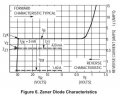From this data, you can see the the impedance at the knee is large; while at the test current, it's much smaller: (Vishay)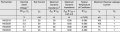The stated power rating is for operation at 25C. You need to derate at higher ambient temperatures.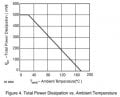#### crutschow

Joined Mar 14, 2008
33,315
I read that the Zener should have 5-100mA of current under load. 5 sounds a bit light
It depends upon the Zener, as shown in the data sheet (Izt).
The ones AK mentioned use a value of 5mA.

•SamR

#### MrChips

Joined Oct 2, 2009
29,791